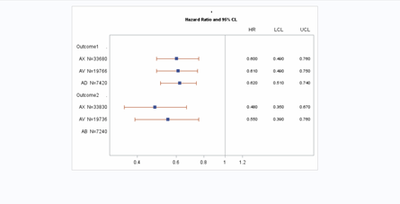## Forest plot modification

``````data forest;
input Study \$1-16 grp OddsRatio LowerCL UpperCL Weight;
format weight percent5. Q1 Q3 4.2 oddsratio lowercl uppercl 5.3;
ObsId=_N_;
OR='HR'; LCL='LCL'; UCL='UCL'; WT='Weight';
if grp=1 then do;

Q1=OddsRatio-OddsRatio*weight;
Q3=OddsRatio+OddsRatio*weight;
lcl2=lowercl;
ucl2=uppercl;
end;
else study2=study;
datalines;
Outcome1      .    .    .   .     .
AC  N=60787     1  0.62 0.53 0.73   1
AX  N=33680     1  0.60 0.49 0.76   1
AV  N=19766     1  0.61 0.49 0.75   2
AD  N=7420      1  0.62 0.51 0.74   1
Outcome2      .    .    .    .     .
AC  N=60738     1  0.51 0.40 0.65   1
AX  N=33830     1  0.48 0.35 0.67   1
AV  N=19736     1  0.55 0.39 0.76   1
AB  N=7240      1  0.50 0.38 0.66   1

;
run;

proc sort data=forest out=forest2;
by descending obsid;
run;

/* Add sequence numbers to each observation */
data forest3;
set forest2 end=last;
retain fmtname 'Study' type 'n';
studyvalue=_n_;
if study2='Overall' then study2value=1;
else study2value = .;

/* Output values and formatted strings to data set */
label=study;
start=studyvalue;
end=studyvalue;
output;
if last then do;
hlo='O';
label='Other';
end;
run;

/* Create the format from the data set */
proc format library=work cntlin=forest3;
run;

/* Apply the format to the study values and remove Overall from Study column. */
/* Compute the width of the box proportional to weight in log scale. */
data forest4;
format studyvalue study2value study.;
drop fmtname type label start end hlo pct;
set forest3 (where=(studyvalue > 0)) nobs=nobs;
if studyvalue=1 then studyvalue=.;
/* Compute marker width */
x1=oddsratio / (1 ** (weight/2));
x2=oddsratio * (1 ** (weight/2));

/* Compute top and bottom offsets */
if _n_ = nobs then do;
pct=0.3/nobs;
call symputx("pct", pct);
call symputx("pct2", 2*pct);
call symputx("count", nobs);
end;
run;

ods listing close;
ods html image_dpi=100 path="." file='sgplotforest.html';
ods graphics / reset width=600px height=400px imagename="Forest_Plot_Vector" imagefmt=gif;

title "";
title2 h=8pt 'Hazard Ratio and 95% CL';

proc sgplot data=forest4 noautolegend;
scatter y=study2value x=oddsratio / markerattrs=graphdata2(symbol=diamondfilled size=10);
scatter y=studyvalue x=oddsratio / xerrorupper=ucl2 xerrorlower=lcl2 markerattrs=graphdata1(symbol=squarefilled size=0);
vector x=x2 y=studyvalue / xorigin=x1 yorigin=studyvalue lineattrs=graphdata1(thickness=8) noarrowheads;
scatter y=studyvalue x=or / markerchar=oddsratio x2axis;
scatter y=studyvalue x=lcl / markerchar=lowercl x2axis;
scatter y=studyvalue x=ucl / markerchar=uppercl x2axis;

refline 1 2 / axis=x;
refline 1 1 / axis=x lineattrs=(pattern=shortdash) transparency=0.5;

xaxis type=log offsetmin=0 offsetmax=0.35 min=0.3 max=1.2 minor display=(nolabel) ;
x2axis offsetmin=0.7 display=(noticks nolabel);
yaxis display=(noticks nolabel) offsetmin=0.2 offsetmax=0.1 values=(1 to &count by 1);
run;

ods html close;
ods listing;``````

Hi everyone,

I am trying to modify the above SAS code but running into a few errors.

- not all data points are displayed.

-I am trying to spell the subgroup completely but adjusting the group length to 1-35 creates issues with the graph

-For the list of groups I am trying to make them aligned to the left and increase the space for the subgroup names with the listed N. Please find a screen shot below1 ACCEPTED SOLUTION

Accepted SolutionsJay54
Meteorite | Level 14

## Re: Forest plot modification

If you have SAS 9.4M3 or later, it is better and easier to use the YAXISTABLE to create forest plots.

3 REPLIES 3

## Re: Forest plot modification

Did you write this code? If not, where did it come from?

## Re: Forest plot modification

It came from SAS forest plot code. I tried to modify their code. https://support.sas.com/kb/42/867.htmlJay54
Meteorite | Level 14

## Re: Forest plot modification

If you have SAS 9.4M3 or later, it is better and easier to use the YAXISTABLE to create forest plots.

Discussion stats
• 3 replies
• 462 views
• 1 like
• 3 in conversation